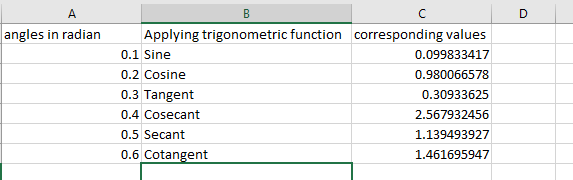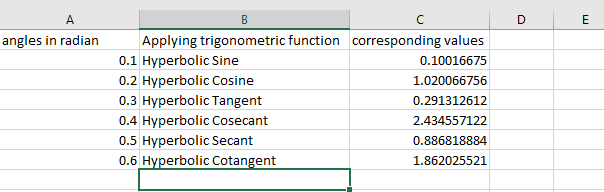# Python | Trigonometric operations in excel file using openpyxl

Prerequisite : Adjusting rows and columns of an excel sheet using openpyxl.

`Openpyxl` is a Python library using which one can perform multiple operations on excel files like reading, writing, mathematical operations and plotting graphs. Let’s see how to perform different Trigonometric operations using openpyxl.

Simple trigonometric functions :

Code #1 : Using simple trigonometric functions in the program.

• =SIN(Number) : Returns the sine of an angle. Number is the angle in radians for which you want the sine.
• =COS(Number) : Returns the cosine of an angle.
• =TAN(Number) : Returns the tangent of an angle.
• =CSC(Number) : Returns the cosecant of an angle.
• =SEC(Number) : Returns the secant of an angle.
• =COT(Number) : Returns the cotangent of an angle.

 `# import openpyxl module ` `import` `openpyxl ` `  `  `# Call a Workbook() function of openpyxl  ` `# to create a new blank Workbook object ` `wb ``=` `openpyxl.Workbook() ` `  `  `# Get workbook active sheet   ` `# from the active attribute. ` `sheet ``=` `wb.active ` ` `  `# set the width of the column ` `sheet.column_dimensions[``'A'``].width ``=` `20` `sheet.column_dimensions[``'B'``].width ``=` `30` `sheet.column_dimensions[``'C'``].width ``=` `20` ` `  `# writing to the cell of an excel sheet ` `sheet[``'A1'``] ``=` `"angles in radian"` `sheet[``'A2'``] ``=` `0.1` `sheet[``'A3'``] ``=` `0.2` `sheet[``'A4'``] ``=` `0.3` `sheet[``'A5'``] ``=` `0.4` `sheet[``'A6'``] ``=` `0.5` `sheet[``'A7'``] ``=` `0.6` ` `  `# mention performing trigonometric operations ` `sheet[``'B1'``] ``=` `"Applying trigonometric function"` `sheet[``'B2'``] ``=` `"Sine"` `sheet[``'B3'``] ``=` `"Cosine"` `sheet[``'B4'``] ``=` `"Tangent"` `sheet[``'B5'``] ``=` `"Cosecant"` `sheet[``'B6'``] ``=` `"Secant"` `sheet[``'B7'``] ``=` `"Cotangent"` ` `  `# The value in cell C1 to C7 is set to a formula  ` `# that calculates values for particular radian. ` `sheet[``'C1'``] ``=` `'corresponding values'` `sheet[``'C2'``] ``=` `'= SIN(0.1)'` `sheet[``'C3'``] ``=` `'= COS(0.2)'` `sheet[``'C4'``] ``=` `'= TAN(0.3)'` `sheet[``'C5'``] ``=` `'= CSC(0.4)'` `sheet[``'C6'``] ``=` `'= SEC(0.5)'` `sheet[``'C7'``] ``=` `'= COT(0.6)'` ` `  `# save the file ` `wb.save(``"simple_trigonometric.xlsx"``) `

Output:Code #2 : Using hyperbolic trigonometric functions in the program.

• =SINH(Number) : Returns the hyperbolic sine of a Number.
• =COSH(Number) : Returns the hyperbolic cosine of a Number.
• =TANH(number) : Returns the hyperbolic tangent of a Number.
• =CSCH(Number) : Returns the hyperbolic cosecant of a Number.
• =SECH(Number) : Returns the hyperbolic secant of a Number.
• =COTH(Number) : Returns the hyperbolic cotangent of a Number.

 `# import openpyxl module ` `import` `openpyxl ` `  `  `# Call a Workbook() function of openpyxl  ` `# to create a new blank Workbook object ` `wb ``=` `openpyxl.Workbook() ` `  `  `# Get workbook active sheet   ` `# from the active attribute. ` `sheet ``=` `wb.active ` ` `  `# set the width of the column ` `sheet.column_dimensions[``'A'``].width ``=` `20` `sheet.column_dimensions[``'B'``].width ``=` `30` `sheet.column_dimensions[``'C'``].width ``=` `20` ` `  `# writing to the cell of an excel sheet ` `sheet[``'A1'``] ``=` `"angles in radian"` `sheet[``'A2'``] ``=` `0.1` `sheet[``'A3'``] ``=` `0.2` `sheet[``'A4'``] ``=` `0.3` `sheet[``'A5'``] ``=` `0.4` `sheet[``'A6'``] ``=` `0.5` `sheet[``'A7'``] ``=` `0.6` ` `  `# mention performing trigonometric operations ` `sheet[``'B1'``] ``=` `"Applying trigonometric function"` `sheet[``'B2'``] ``=` `"Hyperbolic Sine"` `sheet[``'B3'``] ``=` `"Hyperbolic Cosine"` `sheet[``'B4'``] ``=` `"Hyperbolic Tangent"` `sheet[``'B5'``] ``=` `"Hyperbolic Cosecant"` `sheet[``'B6'``] ``=` `"Hyperbolic Secant"` `sheet[``'B7'``] ``=` `"Hyperbolic Cotangent"` ` `  `# The value in cell C1 to C7 is set to a formula  ` `# that calculates values for particular radian. ` `sheet[``'C1'``] ``=` `'corresponding values'` `sheet[``'C2'``] ``=` `'= SINH(0.1)'` `sheet[``'C3'``] ``=` `'= COSH(0.2)'` `sheet[``'C4'``] ``=` `'= TANH(0.3)'` `sheet[``'C5'``] ``=` `'= CSCH(0.4)'` `sheet[``'C6'``] ``=` `'= SECH(0.5)'` `sheet[``'C7'``] ``=` `'= COTH(0.6)'` ` `  `# save the file ` `wb.save(``"Hyperbolic_trigonometric.xlsx"``) `

Output:My Personal Notes arrow_drop_upCheck out this Author's contributed articles.

If you like GeeksforGeeks and would like to contribute, you can also write an article using contribute.geeksforgeeks.org or mail your article to contribute@geeksforgeeks.org. See your article appearing on the GeeksforGeeks main page and help other Geeks.

Please Improve this article if you find anything incorrect by clicking on the "Improve Article" button below.

Article Tags :

1

Please write to us at contribute@geeksforgeeks.org to report any issue with the above content.